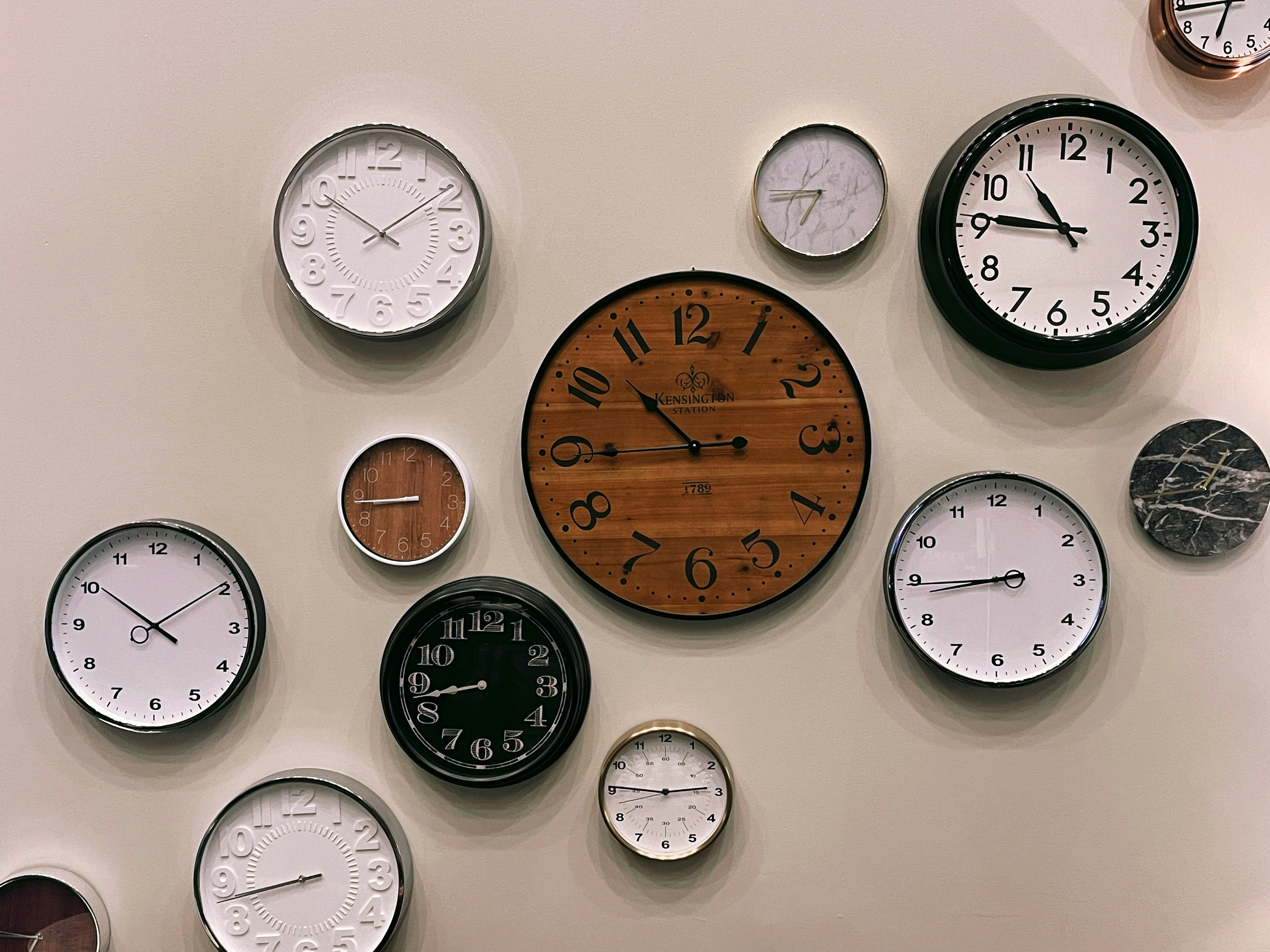# Mastering Date and Time Functions in Notion Formulas - Part Two!

Notion Mar 15, 2023

Managing deadlines and appointments is important for productivity and organization. Notion is a useful tool that can assist in this task, and its built-in formulas can automate many date and time management related tasks.

Previous article in the series featured the first 8 Date and time functions in Notion formulas and how to use those function to build formulas! In this article, we'll explore the remaining date and time functions that you can use in Notion formulas! 🚀

The remaining Date and time functions are:

1. day
2. hour
3. minute
4. month
5. start
6. timestamp
7. year

### 1. minute()

The minute function allows you to extract the minute component of a date and time value. The syntax of the minute function is

`minute(date_time_value)`

For example, if you have a date and time value of "2022-01-01 12:00:00" and you want to extract the minute, you would use the formula `minute("2022-01-01 12:00:00")`, which would return "00".

### 2. hour()

The hour function allows you to extract the hour component of a date and time value. The syntax of the hour function is

`hour(date_time_value)`

For example, if you have a date and time value of "2022-01-01 12:00:00" and you want to extract the hour, you would use the formula `hour("2022-01-01 12:00:00")`, which would return "12".

This simple example shown below combines the now() function with the minute() and hour() functions to show the current time in a 24 hour format!

Using this formula:

As you can see below, our formula works! 🔽

### 3. day()

The day function allows you to extract the day component of a date value. The syntax of the day function is

`day(date_value)`

For example, if you have a date value of "2022-01-01" and you want to extract the day, you would use the formula `day("2022-01-01")`, which would return "01".

### 4. month()

The month function allows you to extract the month component of a date value. The syntax of the month function is

`month(date_value)`

For example, if you have a date value of "2022-01-01" and you want to extract the month, you would use the formula `month("2022-01-01")`, which would return "01".

### 5. year()

The year function allows you to extract the year component of a date value. The syntax of the year function is

`year(date_value)`

For example, if you have a date value of "2022-01-01" and you want to extract the year, you would use the formula `year("2022-01-01")`, which would return "2022".

Similar to the previous example, this simple example shows the combineD use of the day(), month() and year() functions! Note that the day() and month() functions return a value in integers. The day() functions returns a value between 0 to 7, unique for each day of the week starting from 0 at sunday. Similarly the month() function return a value between 0 and 11, uniqe for each month starting from 0 for january.

Formula used:

As you can see below, our formula works! 🔽

### 6. start()

The start function allows you to extract the start of a date and time value, depending on the specified granularity. The syntax of the start function is `start(date_time_value, granularity)`

For example, if you have a date and time value of "2022-01-01 12:00:00" and you want to extract the start of the day, you would use the formula `start("2022-01-01 12:00:00", "day")`, which would return "2022-01-01 00:00:00".

### 7. timestamp()

The timestamp function allows you to convert a date and time value to a timestamp (a number of seconds since the Unix epoch). The syntax of the timestamp function is

`timestamp(date_time_value)**`

For example, if you have a date and time value of "2022-01-01 12:00:00" and you want to convert it to a timestamp, you would use the formula`**  timestamp("2022-01-01 12:00:00")`, which would return "1609459200".

### Conclusion

Now you know about all the Date & Time functions that can be used in Notion formulas! Use these functions in your own Notion space to make it more interactive and feature-rich! Build your own custom formulas to fit your personal needs and unlock the maximum potential of Notion! 💯

Remember to check out the whole Fantastic Formulas series for a complete and comprehensive guide on Notion formulas! ⚡